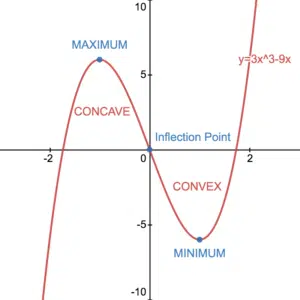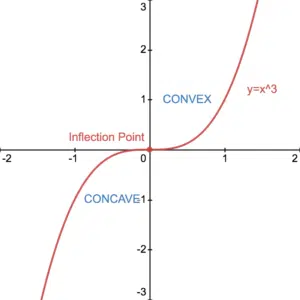# Convex and Concave Functions and Inflection Points

Convex and concave are words that we use to describe the shape or curvature of a curve. Recall from classifying stationary points (see Stationary Points page) that we can find the second derivative of a function by differentiating twice. We also require the second derivative to see if a function is concave or convex at a particular point. A point of inflection is where a curve changes from being concave to being convex or vice versa.

## Convex and Concave FunctionsRecall firstly that solving $f'(x)=0$ for $x$ will identify the locations of the stationary points of the curve $y=f(x)$. Secondly, finding the value of $f”(x)$ at these stationary points allows us to classify the stationary points. That is if $f”(x)<0$, it is a maximum and if $f”(x)>0$, it is a minimum.

It is, however, possible to find the value of $f^{”}(x)$ at any point on a curve, not necessarily stationary points. If at any point on a curve, the second derivative is negative, we say that the curve is concave at that point. Conversely, if the second derivative is positive at any point, we say that the curve is convex at that point. It follows that there is an interval around a maximum that is concave and an interval around a minimum that is convex. See Example 1. The point where a curve changes from being concave to convex or vice versa is known as an inflection point.

Consider the graph of $y=x^2$. The derivative is $\frac{dy}{dx}=2x$ and so the second derivative is $\frac{d^2y}{dx^2}=2$. This is always positive and so the curve is always convex. Similarly for the graph of $y=-x^2$, $\frac{d^2y}{dx^2}=-2$ and so this curve is always concave. It can help to remember that a concave curve has the shape of an actual cave.

## Points of InflectionConsider the graph of $y=x^3$. The derivative is $\frac{dy}{dx}=3x^2$ and the second derivative is $\frac{d^2y}{dx^2}=6x$. The second deriative is 0 when $x=0$, it is positive when $x>0$ and negative when $x<0$. It follows that the point $(0,0)$ is an inflection point.  Also, the curve is concave when $x<0$ and convex when $x>0$. A point of inflection is where a curve goes from being concave to convex or vice versa. This means that the second derivative changes sign. For the curve of $y=x^3$ the point $(0,0)$ is a stationary point as well as a point of inflection. However, an inflection point doesn’t have to be a stationary point as we can see in the section above. Also see Example 2. It is the fact that $f”(x)=0$ and $f”(x)$ changing sign that makes a given point one of inflection.

## Convex, Concave and Inflection Examples

Determine whether the curve of $y=x^2-e^x$ is convex or concave at the points with $x$-coordinates $x=0$ and $x=1$.

Determine the the inflection point of the curve of $f(x)=x^2\ln(x)-\frac{5}{2}x^2$. Hence, determine the concave and convex regions. Is the point of inflection also a stationary point?